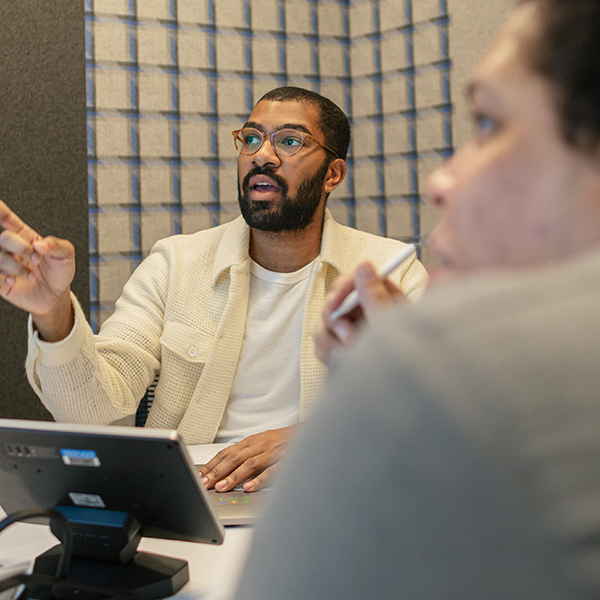# C++ Programming – From Scratch to Advanced

Learn C++ from the basics to the advanced concepts.

Language: english

Note: 4.5/5 (322 notes) 27,330 students

Instructor(s): Sujithkumar MA

Last update: 2022-06-24

## What you’ll learn

• C++
• Object Oriented Programming
• Programming

## Requirements

• No, Anyone who wants to start coding can learn

## Description

In this course, C++ Programming from Scratch to Advanced, You’ll learn everything to make you confident on C++ concepts.

The primary course objectives will be

1. Understanding the fundamentals of programming.

2. Analyzing the range of each and every datatype and understanding how they’re stored in the memory.

3. Understanding how a C++ Program gets executed and converted into object code.

4. Implementing high level programming constructs in an easy manner in c++

5. Understanding the importance of addresses and pointers.

6. Knowing how to use pointers.

7. Understanding the significance of Objects, Classes and Inheritance and knowing how to implement them.

8. Understanding the differences between Access Modifiers and Data Modifiers and how they influence the classes and their data members.

Features:

1. The trickier concepts of C++ like Pointers, Inheritance, Pointer to an array, Pointer to a function, Multilevel Inheritance are clearly explained.

2. The course has video content from the scratch of programming. So, this suits even if someone is no exposed to any of the programming languages.

3. Solving some standard programming problems.

4. The concept of Recursion is clearly interpreted.

5. The concept of Bitwise Operators is explained by performing Decimal to Binary conversions.

6. The usage of constructors is explained.

Note: For all the lectures, the lecture notes and the source codes have been uploaded.

## Who this course is for

• Beginner Coding Aspirants

## Course content

• Understanding all the basic components of a C++ Code
• Introduction
• Components in a C++ Program
• Writing our first program in C++
• The Execution flow of a C++ Program – Compiler, Assembler, Linker
• Basic I/O
• Analyzing the types of variables
• Datatypes and Variables in C++
• Coding Examples – Datatypes
• Calculating the Range of Integers
• Calculating the Range of Floating Point Numbers
• Calculating the Range of Double Precision Floating Point Numbers
• Why using namespace std ?
• Modifiers – Signed, Unsigned
• Short Integers
• Arithmetic Operators
• Different types of Assignment Operators
• Relational operators
• Comments, Increment and Decrement Operators
• Working of Logical operators
• Precedence and Associativity
• Working of Bitwise Operators
• BItwise AND
• Bitwise OR
• Bitwise XOR and Bitwise NOT
• Bitwise Left Shift and Right Shift Operators
• High Level Programming Constructs
• If-else statements
• Introduction to loops
• Working of a for loop
• An example for for loop
• While loops, Difference between While Loop and For Loop
• Importance of do while loops
• Example – Generating Fibonacci Series in C++
• The concept of Arrays and Matrices
• Introduction to Arrays and Array Indexing
• An Example program using arrays
• Algorithm to reverse the contents of an array
• Multidimensional arrays in C++
• Working of Nested For Loops
• Generate a 2D Matrix in C++
• Functions and Structures
• The terminologies of a function
• Example 1 – Function without arguments, without return statement
• Example 2 – Function with arguments, with return statement
• Example 3 – A function is a processor
• Importance of Structures in C++
• Creating structures in C++ – Code
• Recursion
• Understanding Recursion with an example
• Example 2 for Recursion – Tracing the output
• Tricks to write recursive functions !
• String object in C++ and Character Arrays
• Using character arrays to create Strings
• Problem of using cin for strings
• get() function for strings
• String object in C++
• Pointers
• The concept of Pointers and Addresses
• Syntax to create pointers and referencing
• Understanding the crucial aspects of pointers and addresses
• Types of Pointers
• Representation of an array in the memory
• Pointer to an array
• Pointers passed as arguments – call by reference
• Dynamic Memory Allocation in C++
• Importance of Dynamic Memory Allocation
• Importance of calloc and free
• Object Oriented Programming
• OOP – Classes and objects with real world examples
• Identity, Attributes and Behavior of an object
• Implementation of an OOP Based Car example
• Importance of Getters and Setters in C++
• Access Modifiers and Data hiding
• All about Constructors in C++
• Inheritance and Destructors
• Inheritance – Base Class, Derived Class
• Implementation of derived classes in C++
• Inheritance Access Controls & Public Access
• Inheritance Access Controls & Private, Protected Access
• Multiple Inheritance and Multilevel Inheritance
• Function Overriding in C++
• Resources
• Bonus Lecture 1
• Bonus Lecture 2
• Update – STL
• Cpp STL – 1
• Cpp STL – 2
• Final MCQ Test
• Final C++ Quiz